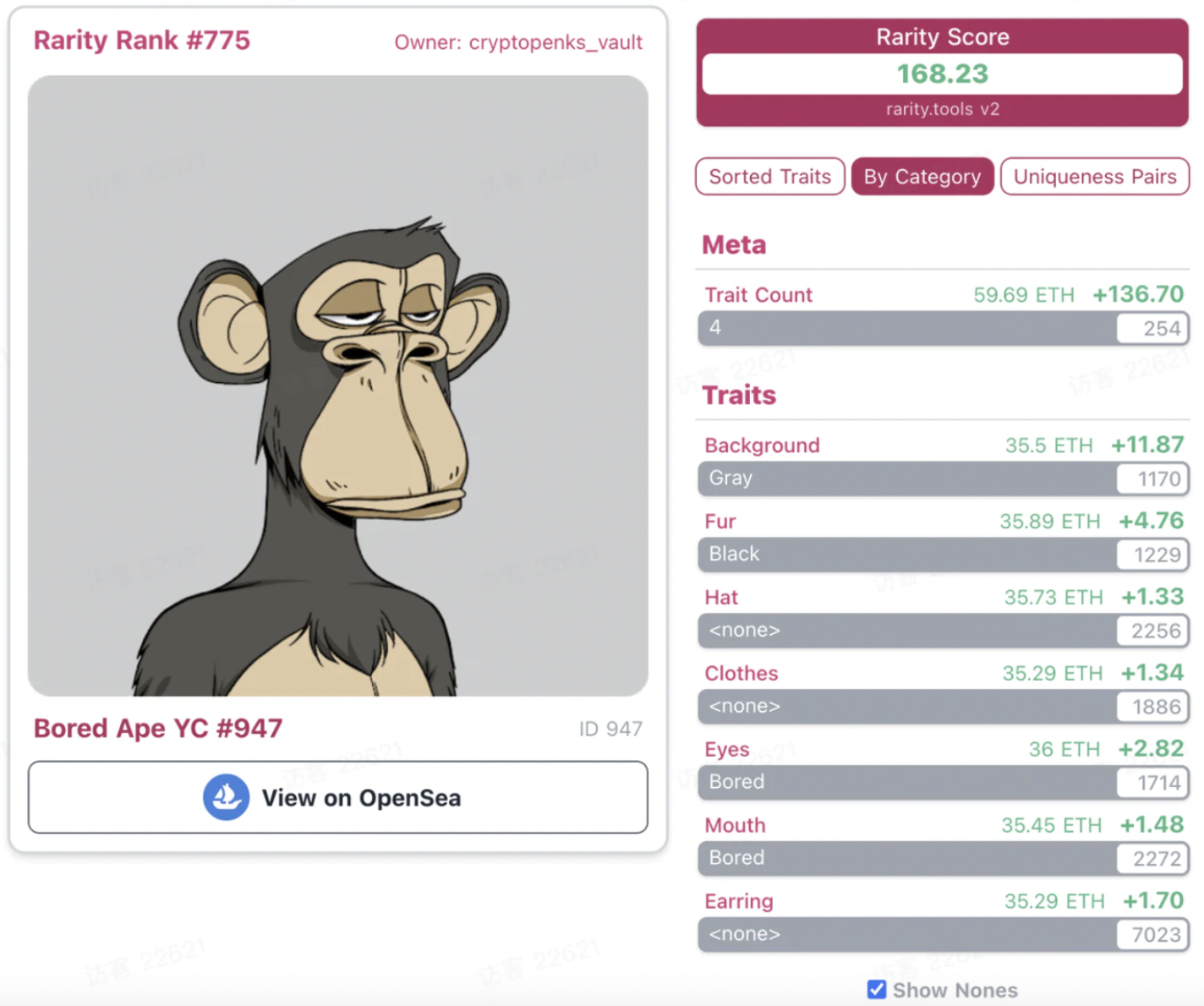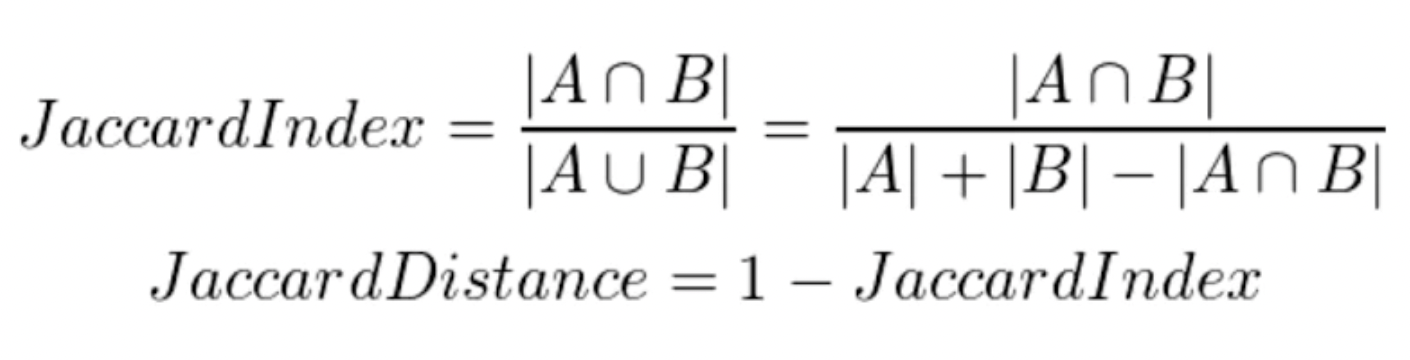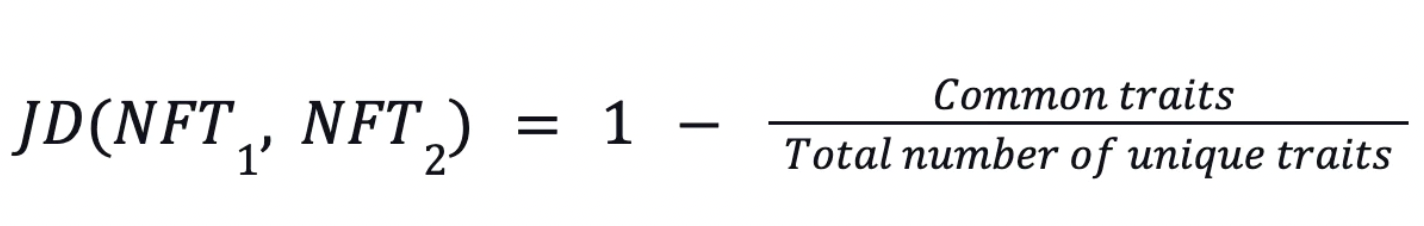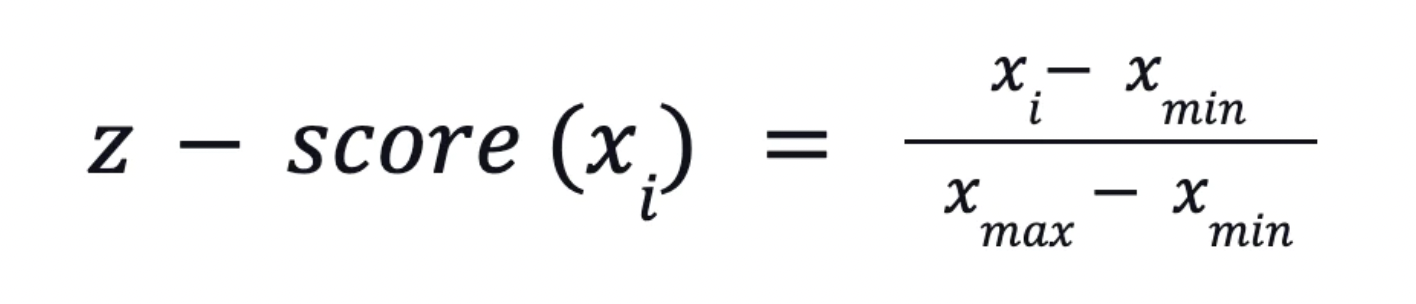# NFTGo Rarity Model

Rarity model created by NFTGo.io

Essentially, the rarity of an object depends largely on its differences from other objects: the greater the difference is, the more special and rarer this object will be. Quantifying the combined difference between an object and other things within the group reflects the object’s rarity. Based on this logic, NFTGo.io uses a statistical approach called Jaccard distance to evaluate the real rarity of NFTs. An explanation of the specific algorithm can be found below.

## What is Jaccard distance?

NFTGo.io’s innovative rarity evaluation model goes beyond the mainstream approaches employed by products like rarity.tools. The common way of approaching NFT rarity is flawed and it has a low accuracy in some cases. An example of an inaccurate prediction from these models is the rarity score for Ape #947 from the Bored apes club. Rarity.tools gave a rarity score of 168.23. Even though the NFT does not seem to have any unique attributes.The reason is that these models naively add up the traits and the frequency of occurrence for each of the traits. This simple approach doesn’t take into account how each trait contributes to an NFT’s uniqueness. This is why NFTGo.io has created a complex model that takes a more scientific approach to estimating an NFT’s rarity.

Jaccard distance is a metric for measuring similarities between two sets of data. In our case, the Jaccard distance takes into account the similarities between NFTs based on their attributes and assigns a rarity score ranging from 0 to 100.The distance between two data points, in this case, is equal to the number of similar attributes divided by the total number of their attributes. This number is subtracted by one to get the Jaccard distance.

We see how this plays out in different NFTs. Our model employs this algorithm to quantitatively measure the similarities between two NFTs. This opens up the possibility for us to estimate an NFT’s rarity scientifically.

## Deeper Dive

This approach measures the overlap between two finite sets. The higher the overlap, the lower the Jaccard distance. By understanding the similarities across all the NFTs in a collection, we can gauge how rare an NFT is. For evaluating an NFT’s rarity, we compare it to all the other NFTs in the collection. For each NFT pair, we take the Jaccard distance and normalize the results to get the optimal score.

The process of calculating an NFT’s rarity mainly contains 4 steps:

Step 1 - Dividing the number of similar traits by the total number of unique attributes. Then, subtracting the results from one. (We repeat this process for all the NFT pairs)Step 2 - Taking the average of all the resultsStep 3 - Normalizing the resultsStep 4 - Multiplying the z-score by 100